## Friday, 30 March 2018

### Another Three Cones and a Sphere

In my previous post I solved a geometry problem involving three cones and a sphere. This time, I solve the same problem, with one small difference: the bottom of the sphere is above the elevation of the base of the cone. What is the sphere's radius, R_sphere, in terms of h and R_cone? And how high is the sphere above the bases of the cones? (Bonus: what additional conditions are necessary if R_sphere = R_cone?)A sphere resting on three identical cones with mutually tangent bases.

Since we know that the sphere is suspended above the ground in this problem, we can conclude that the height of the cone must be at least 0.845299 times the radius of the cone for this scenario to work (to see where that number comes from, view my post on a similar problem where the sphere did touch the ground).

First, let's look at the vertical section through one cone and the sphere at the point of contact.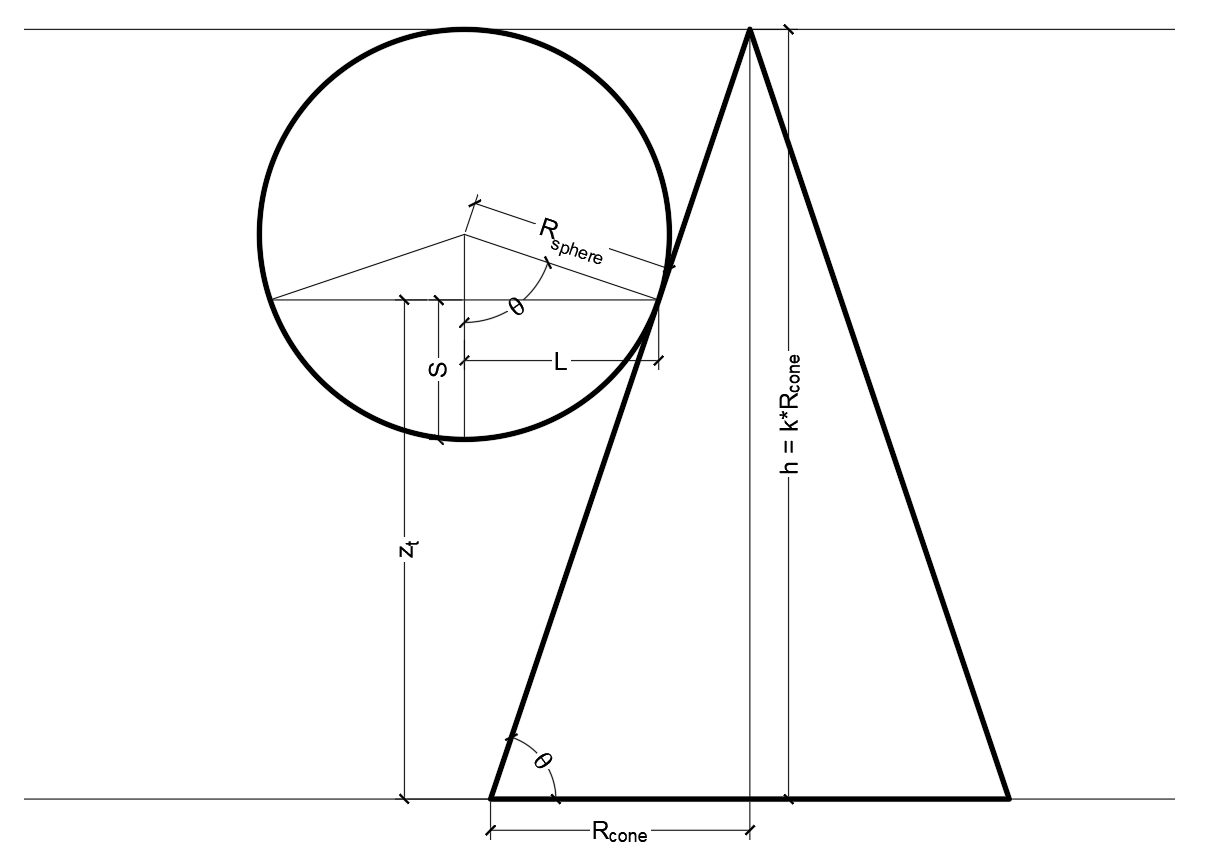Cross-section through the sphere and one cone at the contact point.

We will relate the height of the cone to radius of the cone such that h = kR_cone (k = tan[θ] > 0.845299). We will also call the height above the base where the contact occurs z_t. The radius of the horizontal circle through the three contact points on the sphere will be L. The depth of the sphere below the contact will be S, equal to the sagitta of the arc with radius R_sphere through an angle 2θ and having chord length 2L.

From this diagram we can come up with a few relationships: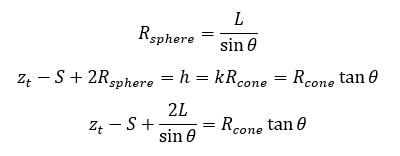Adding in the equation of the sagitta S = R_sphere*versin[θ], substituting and simplifying, we get: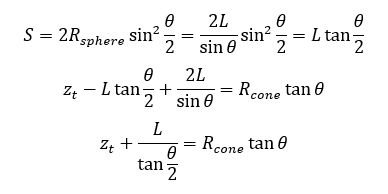We need some more information. Let's now look at the plan view, starting with the level of the cones' bases.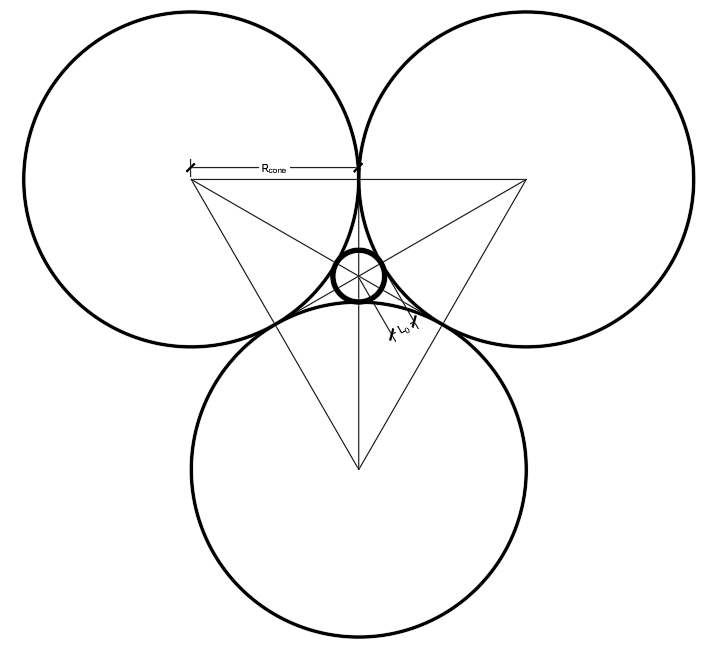Horizontal section view of the mutually tangent circular bases of the three cones, and the inner Soddy circle between them.

The maximum size of circle that can be inscribed in the space between the three mutually tangent circular bases is sometimes called an inner Soddy circle. Its radius can be calculated from Descarte's Theorem. We'll call that radius L_0, which works out to: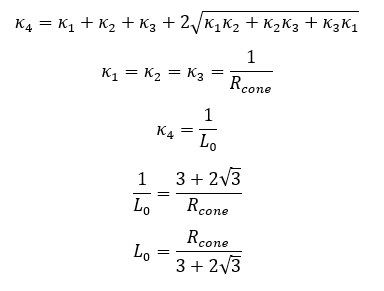Radius of the inner Soddy circle between the mutually tangent circular bases of the three cones.

Let's look at a horizontal cut through the contact points on the sphere now.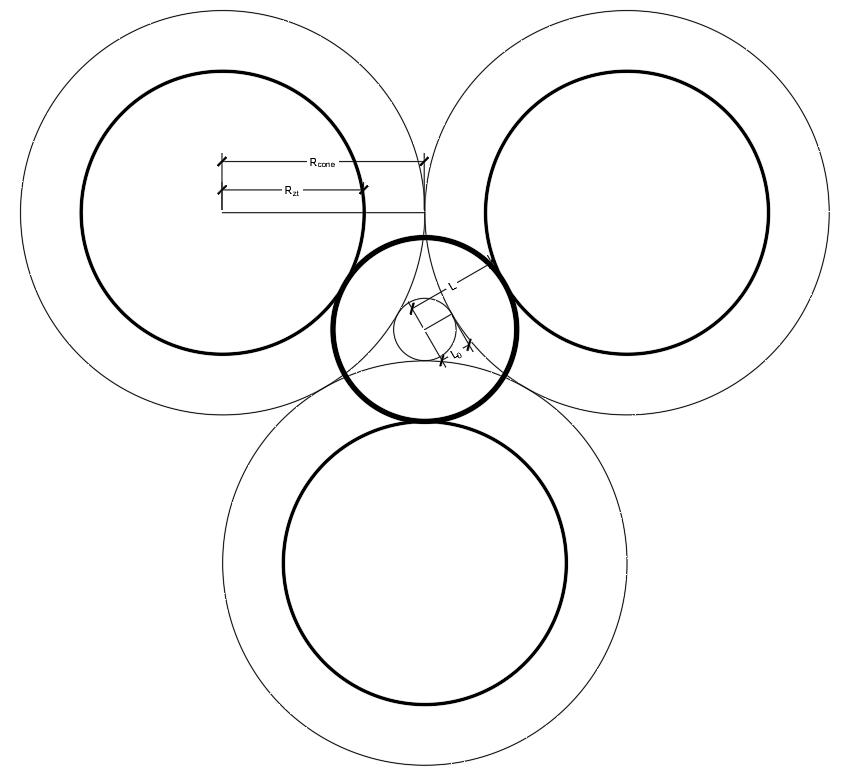Horizontal section view of the cones and sphere at the contact points The cones bases and inner Soddy circle are also shown for reference.

The radius of the cone decreases linearly with height, from R_cone at the base to 0 at the vertex. At the height z_t above the base, the cone radius is R_zt = R_cone - z_t / k. Meanwhile, the radius of the circle between the cones increases linearly, from L_0 at the base to L_0 + R_cone at the vertices. At the point of contact between the sphere and the cones, L = L_0 + z_t / k.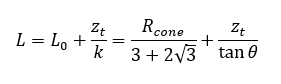Radius of the horizontal circle through the three contacts between the sphere and the cones.

Now let's start by solving for L, the radius of the circle through the three contact points. From the horizontal section we get: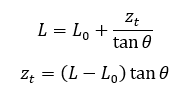Equation 1

and from the vertical section we get: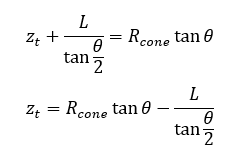Equation 2

Equating the two, we get: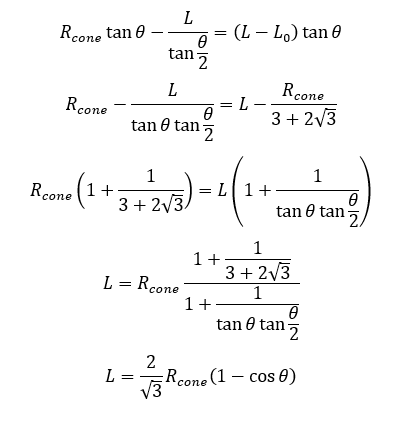Solution for L

Now that we have L, we can go back and solve for everything else. z_t, the height of the contact points above the base is: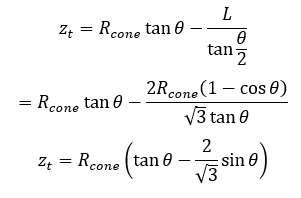Solution for z_t

The radius of the sphere is: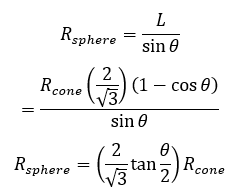Solution for R_sphere

The sagitta, S (depth of the spherical cap below the contact points with the cones) is: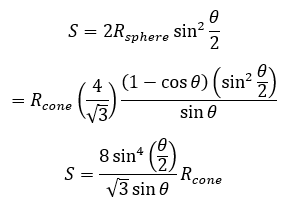Solution for S

The height of the bottom of sphere above the ground is the height of the cone less the diameter of the sphere: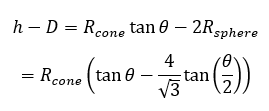Solution for h - D = height of the sphere's bottom above the ground

And for completion, the angle θ is given by: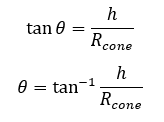Angle θ related back to height and radius of the cone.

Finally, let's see what that looks like in tabulated and graphical forms. I use normalized values here as follows: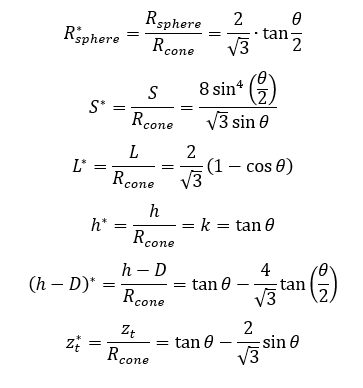Normalized values of R_sphere, S, L, h, (h-D), and z_t

These normalized lengths are tabulated below: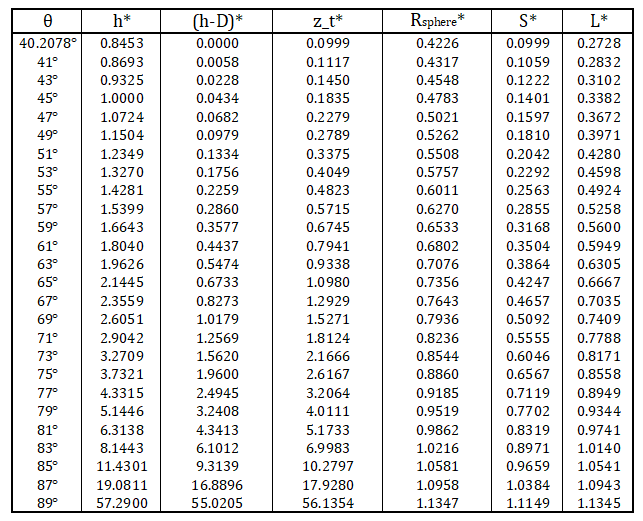Tabulated normalized lengths for the three-cones-and-a-sphere problem.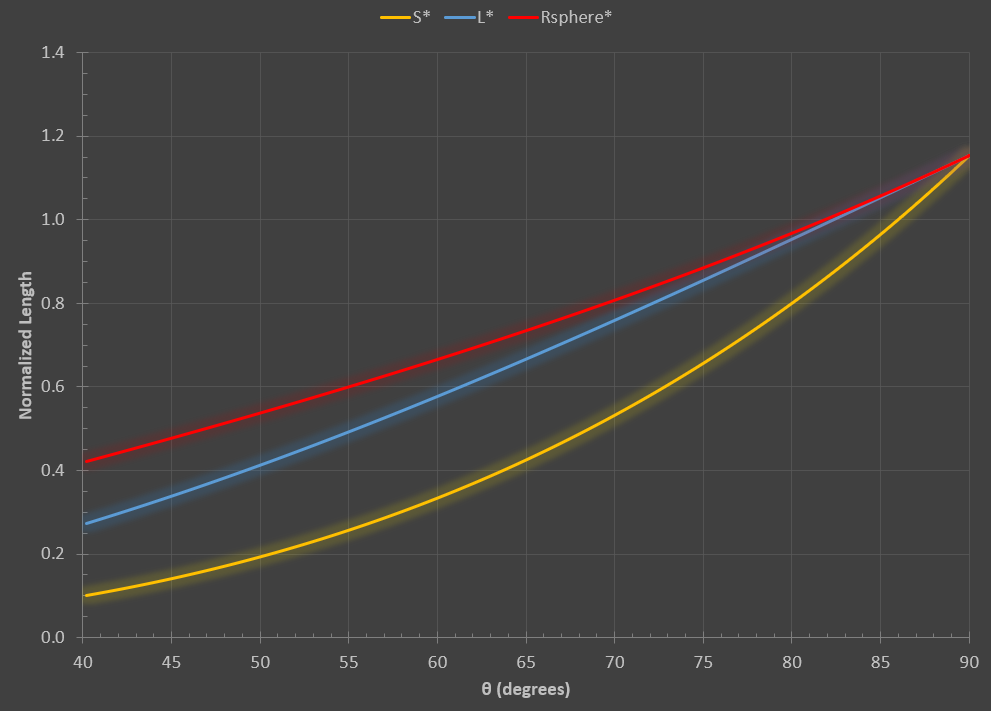Normalized sagitta S*, radius of the circle through the contact points L*, and radius of the sphere R_sphere*

S*, L*, and R_sphere* all converge on the value of 2 / sqrt (about 1.1547) as the angle θ approaches 90 degrees.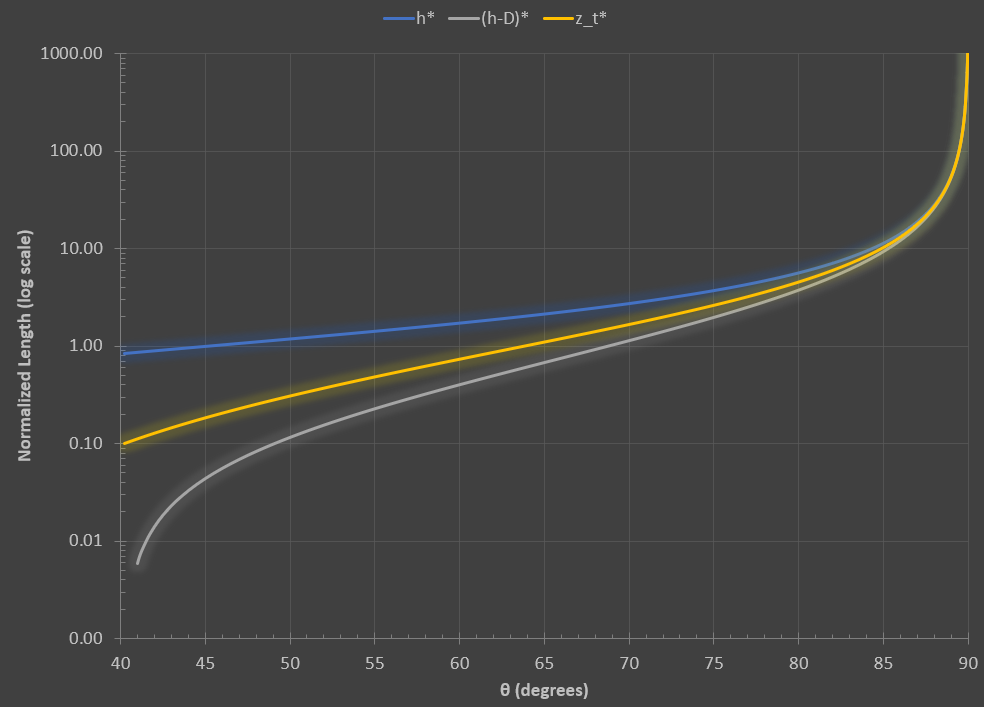Normalized cone height h*, height to bottom of sphere (h-D)*, and height to sphere-cone contact points z_t*

Beyond about 85 degrees, h*, (h-D)*, and z_t* all begin to grow quickly because tan[θ] approaches infinity.

Bonus: For R_sphere = R_cone, solve R_sphere* = 1: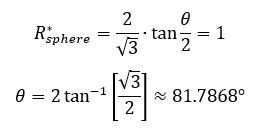Solution to the specific case where R_sphere = R_cone

1.2.